Symmetric and skew symmetric matrices

Chapter 3 Class 12 Matrices
Concept wise

For any square matrix A,

(A + A’) is a symmetric matrix

(A − A’) is a skew-symmetric matrix

Let’s first prove them

(A + A’) is a symmetric matrix

For a symmetric matrix

X’ = X

So, we have to prove

(A + A’)’ = (A + A’)

Solving LHS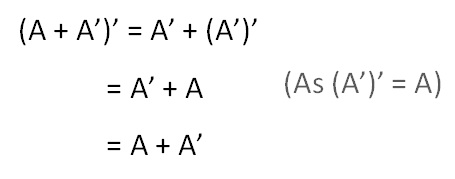Therefore,

(A + A’)’ = A + A’

So, A + A’ is a symmetric matrix

## (A − A’) is a symmetric matrix

For a skew symmetric matrix X

X’ = −X

So, we have prove

(A − A’)’ = − (A − A’)

Solving LHS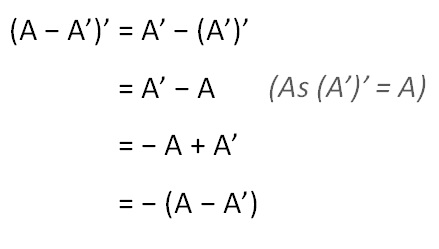Therefore,

(A − A’)’ = − (A − A’)

So, A − A’ is a skew symmetric matrix

Now,

Let’s write matrix A as sum of symmetric & skew symmetric matrix

(A + A’) + (A − A’) = 2A

So,

1/2 [(A + A’) + (A − A’)] = A

1/2 (A + A’) + 1/2 (A − A’) = A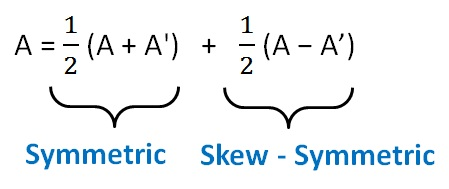Here,

1/2 (A + A’) is the symmetric matrix

&   1/2 (A − A’) is the symmetric matrix

Let’s take an example,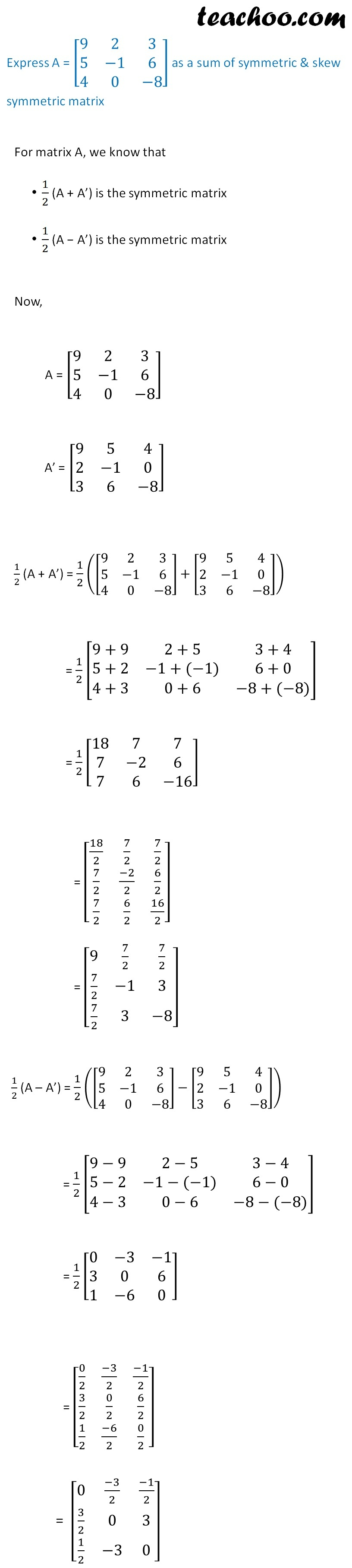Let’s check if they are symmetric & skew-symmetric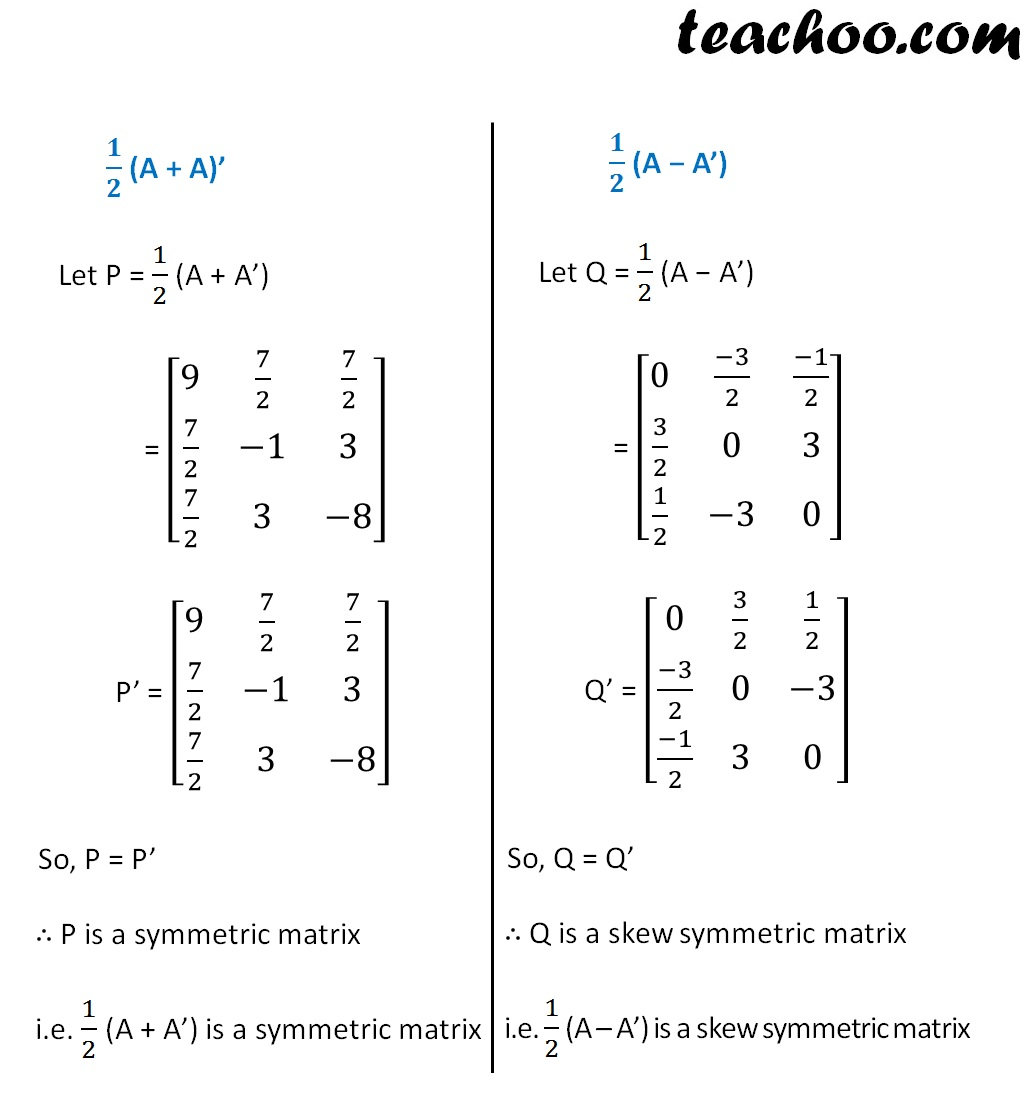Learn in your speed, with individual attention - Teachoo Maths 1-on-1 Class